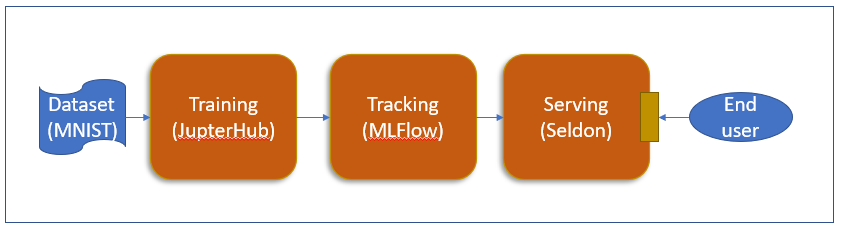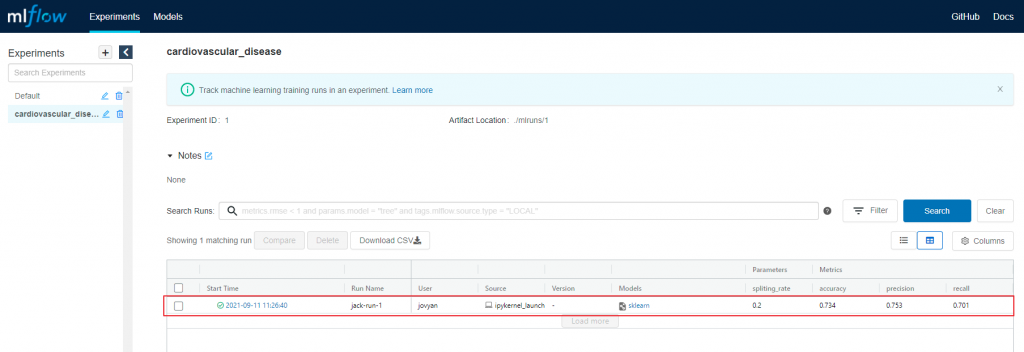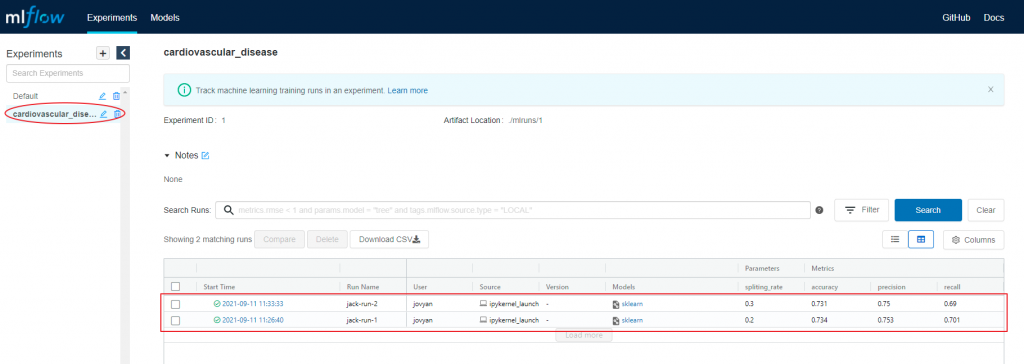#DAY 13
0
AI & Data

## 心血管疾病notebook使用MLFlow做記錄https://github.com/masonwu1762/ithome-ironman

• notebook: cardiovascular_disease_prediction_notebook_mlfow.ipynb
• dataset: cardio_train.csv

``````!pip install mlflow
``````

``````# Import mlflow
import mlflow
import mlflow.sklearn
``````

``````mlflow.set_tracking_uri("http://172.23.180.10:30534")
``````

``````mlflow.set_experiment("cardiovascular_disease")
``````

``````with mlflow.start_run(run_name="jack-run-1"):
mlflow.log_param('split_rate', split_rate)

mlflow.log_metric('precision', precision_score(y_test, predict))
mlflow.log_metric('recall', recall_score(y_test, predict))
mlflow.log_metric('accuracy', accuracy_score(y_test, predict))

mlflow.sklearn.log_model(model, artifact_path="xgb-model")
``````

``````split_rate = 0.2
``````

``````with mlflow.start_run(run_name="jack-run-1"):
````````````split_rate = 0.3
``````

``````with mlflow.start_run(run_name="jack-run-2"):
``````